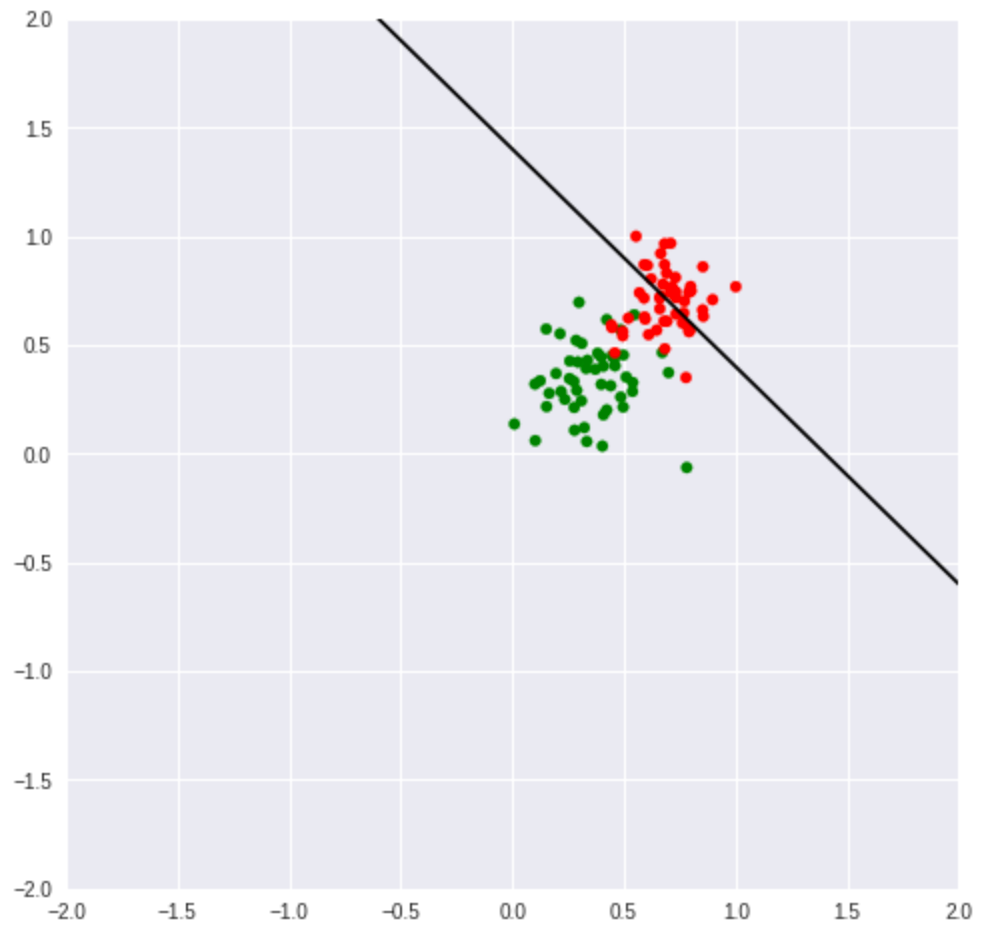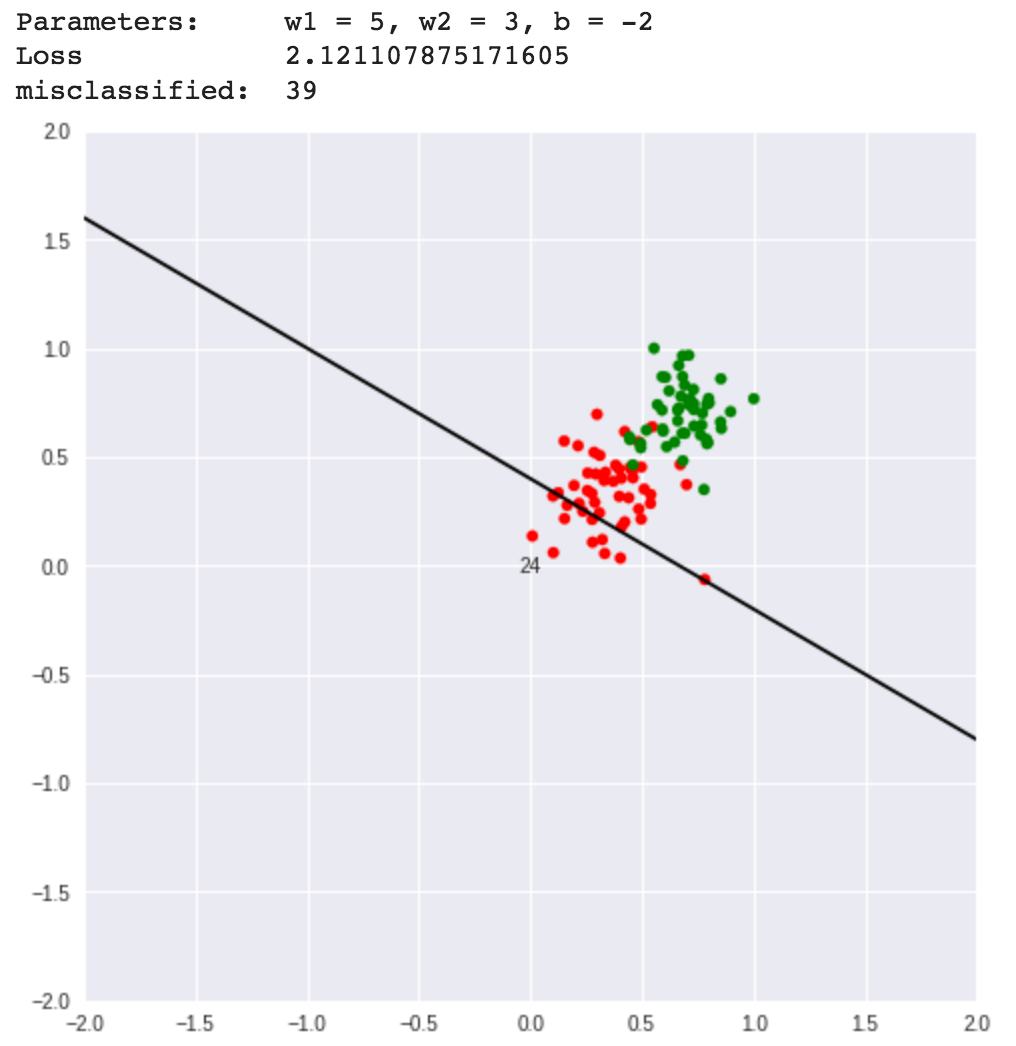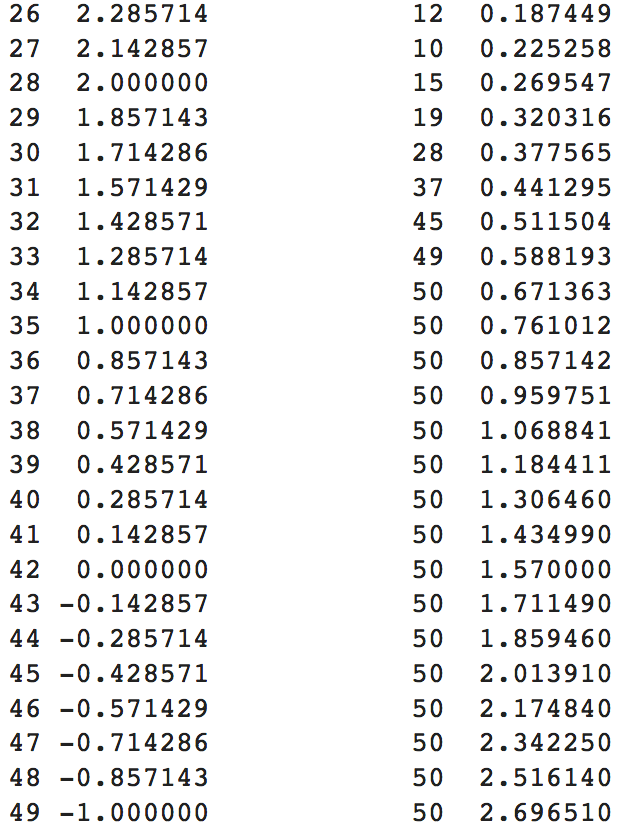# Intuitive guide to neural networks — Part2

#### Perceptron optimization, learning, gradient descent

In the last article we understood what perceptrons are and how to model a binary classification task using them, the importance of parameters (weights w1, w2 and bias b) and solved the problem of classifying outputs of an AND gate.

But we manually picked the values for parameters and we left off with the note that we need an automated approach or an algorithm which when fed the data would find out the values for these parameters. Here is the problem statement in short which we set out to solve in the last blog, given the data set of university acceptance based on test score and grades, we need to find the straight line decision boundary which separates the student who were accepted from those who were rejected.

Thought worm : What if the relationship between inputs and the output is complex and they cannot be separated by a straight line?

Here are the steps to find the optimal values for parameters w1, w2 and b.

#### Step 1: Start with a assigning a random value for w1, w2 and b

Assign random initial values for w1, w2 and b and see how well it separates the data.

Here is the notebook cell, make a copy and try running it yourself on cloud.Left: w1 = 5, w2 = 3, b = -2 . Right: w1 = 1, w2 = 1, b = -1.4

The above images shows how well the straight line defined by 2 random set of parameters separate the data.

But which one of the above lines classify the data better ? In other words, which one of the above two set of parameters best classify the data?

That brings us to ways of evaluating how good or how bad a given set of parameter classifies the data.

#### Step 2: Calculate the error or loss

Think about this? What could be the possible ways to evaluate how well or how bad a set of parameters classifies the data?

One way is to find the number of correctly classified or wrongly classified data points. Let’s see the number of misclassifed or wrongly classified data points for above two set of parameters .

The points in blue represents all the data points which are wrongly classified by the straight line.

So the number of wrongly classified points by parameters (1, 1, -1.4) is 24, and parameters (5, 3, -2) is 39. So definitely the first set of parameters are better. Here is the link to the notebook cell, try running it yourself by changing the values of w1, w2 and b.

Though the number of wrongly classified points helps us compare the effectiveness of different sets of parameters, its not sufficient to optimize the values of w1, w2 and b. We need a continuous value error function which captures more information about how badly the straight line is classifying the points.

We have 100 student records for the university admission data, how about we use a metric which is a function of both the ground truth label and the prediction.

Consider the ground truth label to be y and the prediction to be y^ (spelled has y hat), the goal here is to reduce the difference between y and y^ for all the examples, that is to choose values for parameters w1, w2 and b which reduces (y – y^) . How about we choose the popular squared error function?

`error = (1/2) * (y - y^)²`

But the squared error function doesn’t work well for classification tasks. In the next section we’ll see an illustration on how it fails to encapture the degree of correctness of the classification.

Here is the algorithm to find the cumulative error using the squared error function.

`cumulative_error = 0 initialize w1, w2, and b.`
`For i range(total_records):   1. find prediction y^   2. find the error e = (1/2) * (y - y^)   3. Sum up the errors, cumulative_error = cumulative_error + e `
`# Find the average for the cumulative errorcumulative_error = cumulative_error / total_num_records`

The cumulative error is called the loss of the network, the loss function is represented by L(y, y^) .

Let’s take the 3 sets of parameters we considered in the above examples and calculate the loss and compare them, the better set of parameters should have the least loss.Plots for parameters with their number of wrongly classified points and the loss

I know what you are thinking right now, the squared error loss seems to reduce with the reduction in number of wrongly classified points and hence we can use it as an error function. Isn’t it? Right guess, but it doesn’t quite work like that when we take a deeper look at it. Let’s again generate the above table, but this time with more points. Let’s keep values of w2 and b constant and generate bunch of values for w1 and obtain the number of wrongly classified points along with the loss value.

If the squared error function were to rightly capture the correctness of the classification the loss should reduce with reduction in number of wrongly classified points. Let’s see what happens.The number of classified points with its loss for various values of weight1

Take a look at those marked points circle in red, the value of loss is at its least value of 0.095604 when the number of wrongly classified points is 20.

But when the number of wrongly classified points are 10 the value of loss is expected to reduce compared to what it was when it misclassified 20 points, but instead the loss increases gradually to 0.22 .

This is like an intuitive and empirical proof that the squared error function doesn’t actually encapture the goodness of a classifier, it basically tries to fit a regression line and fails to understand that the outputs 1 and 0 are the values which just indicates the category of data and these are not continuous valued outputs. In the next blog we’ll discuss more about the loss functions and optimization techniques that can be used in classifiers.

But how do we optimize the parameters to fit a straight line which can best classify these two classes of data?

#### The optimization technique

Phew, finally we’ve reached the climax. The issue with the squared error function was that it even took the large error values into consideration from the correctly classified points. We need to extract as much information as we can only from the wrongly classified points.

There are two cases where the straight line boundary would be wrong,

Case 1: When the straight line is too much into the red points area.

• This is the case where lot of red point are misclassified and almost none of the green points are wrongly classified.
• The perceptrons parameter set characterizing the straight line are ( 5, 3, -2 ).
• The prediction of perceptron in this case for all the misclassified red points is 1, but their actual label is 1.
• In this case the line has to be pulled back towards the green cluster.
• This can be done by updating the parameters by subtracting the values of these wrongly classified points with them.

Here is the sequence of steps to be followed to optimize the parameters for this case,

`initialize w1, w2 and b`
`For all wrongly classified red points(that is when y = 0 and y^ = 1)`
`   # x1 represents the test score of misclassified red point   w1 = w1 - (a small number) * x1      # x2 represents the y-axis value of misclassified red point   # x2 represents the grades.   w2 = w2 - (a small number) * x2`
`   # update bias    b = b - (a small number)`

To make sure that the update to parameters w1, w2 and b are not very large, we multiply the values with a small number before the update. This small number is called learning rate and is represented by value α (alpha). Usually the learning rate will of order 0.1 to 0.0001. The convergence of the parameters to optimal values slows down with decrease in learning rate. But the large value of learning rate would also cause some problems. A reasonable value for learning rate has to set by trial and observation, the learning process doesn’t help you obtain a practical learning rate, such values are called hyper parameters.

Here is the snippet for updating parameter for the first case,

Let us run one iteration of the optimization and see whether the classifier gets better. The number of wrongly classified points should reduce if the optimization is effective. Here are the results before and after the classification,

The first plot corresponds to perceptrons prediction before the optimization, it wrongly classified 39 data points with its initial set of parameters

But after the optimization the performance significantly improves and the number of wrongly classified points reduces to just 6.

Here is the link to the notebook, make a copy, run it yourself and play around. Try running with various values of learning rate. With decrease in learning rate the optimization algorithm has to be run multiple times on the parameters.

Below are series of images which shows the state of the parameters and thus the classification after one run of optimization for various values of learning rates.

Don’t assume that the optimization becomes less effective with smaller learning rates, the optimization just gets slower and has to be run multiple times for parameters to reach the optimal value. Too small a value for learning would make the training slower and you may have to run the optimization function many number of times.

Case 2: When the straight line is too much into the green points area.

• This is the case where lot of green point are misclassified and almost none of the red points are wrongly classified.
• The perceptrons parameter set characterizing the straight line are 1, 1, -1.5.
• The prediction of perceptron in this case for all the misclassified green points is 0, but their actual label is 1.
• In this case the line has to be pushed back towards the red points cluster.
• This can be done by adding the values of these wrongly classified points to the parameters.

Here is the sequence of steps to be followed for this case,

`initialize w1, w2 and b`
`For all wrongly classified green points(that's when y = 1and y^ = 0)`
`   # x1 represents the test score in the university admission data   w1 = w1 + learning_rate * x1      # x2 represents the grades.   w2 = w2 + (learning_rate) * x2`
`   # update bias    b = b + (learning_rate) `

Notice the only difference compared to the previous case, we need to slowly increase the values of the parameters.

Here is the snippet for updating parameter for the second case,

This time let us use a small learning rate of value 0.0001and run the optimization 10 times. In the animation below you can see how the classifier gets better after each run of the optimization algorithm.

Here is the notebook cell with the code for solving this case, as I did mention earlier we are running the training for 10 iterations, and when you run the code from the notebook cell you’ll see that the plot containing the decision boundary (the straight line classifying the points) gets updated after each round of training, run it with various values for learning rate and check it out for yourself.

#### The final solution

We need to just combine the solutions for case 1 and case 2 to obtain the final solution,

Again, we have set a small learning rate and running the iterations for 100 times. Here is the link to notebook cell, try running with various values for parameters w1, w2 and b. You’ll see that the parameters are optimized in a way that it classifies the points more accurately.

This concludes the blog, along with its prequel we went through the journey of building a binary classifier with a linear decision boundary. In the next few blog posts we’ll see how to solve more complex problems using Deep learning. Here is the link to the notebook containing all code samples used in the blogs. As usual, till then, happy coding !Intuitive guide to neural networks — Part2 was originally published in AI India on Medium, where people are continuing the conversation by highlighting and responding to this story.

Source: Deep Learning on Medium